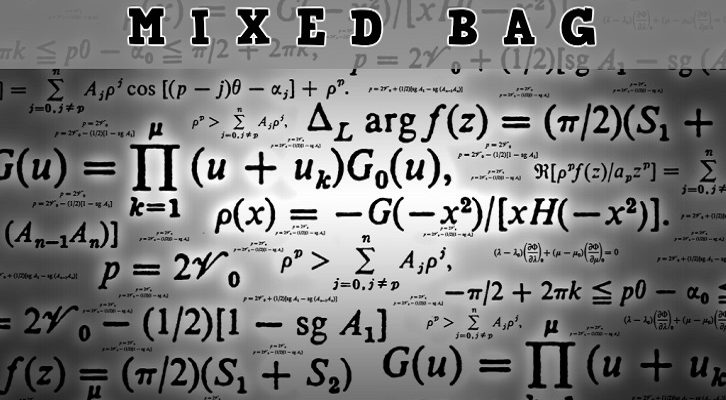# CAT Functions, Onto functions

Question
How many onto functions can be defined from the set A = {1, 2, 3, 4} to {a, b, c}?
1. 81
2. 79
3. 36
4. 45
Answer: Choice (C)
Explanatory Answer:
First let us think of the number of potential functions possible. Each element in A has three options in the co-domain. So, the number of possible functions = 34 = 81.
Now, within these, let us think about functions that are not onto. These can be under two scenarios
Scenario 1: Elements in A being mapped on to exactly two of the elements in B (There will be one element in the co-domain without a pre-image).
Ø  Let us assume that elements are mapped into A and B. Number of ways in which this can be done = 24 – 2 = 14
o   24 because the number of options for each element is 2. Each can be mapped on to either A or B
o   -2 because these 24selections would include the possibility that all elements are mapped on to A or all elements being mapped on to B. These two need to be deducted
Ø  The elements could be mapped on B & C only or C & A only. So, total number of possible outcomes = 14 * 3 = 42.
Scenario 2: Elements in A being mapped to exactly one of the elements in B. (Two elements in B without pre-image). There are three possible functions under this scenario. All elements mapped to a, or all elements mapped to b or all elements mapped to c.
Total number of onto functions = Total number of functions – Number of functions where one element from the co-doamin remains without a pre-image – Number of functions where 2 elements from the co-doamin remain without a pre-image
ð  Total number of onto functions = 81 – 42 – 3 = 81 – 45 = 36
Answer Choice (C)